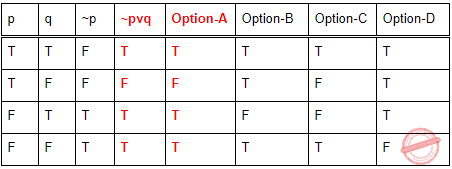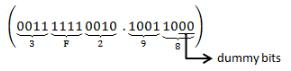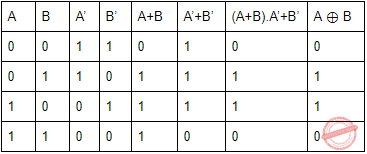## UGC NET CS 2011 June-Paper-2

 Question 1
Any integer composed of 3n identical digits divisible by
 A 2n B 3n C 5n D 7n
Engineering-Mathematics       Combinatorics
Question 1 Explanation:
Identical digits means similar(same) digits.
Any integer composed of 3n identical digits.
For example n=1, 3n= 31=3 which means three identical digits (111,222,333,444 and so on)
n=2, 32 =9 which means nine identical digits (111111111, 222222222 and so on)
The above numbers are is divisible by 3
111 is divisible by 3.
222, 333 and 444 are multiple of 3
 Question 2
The circumference of the two concentric disks are divided into 100 sections each. For the outer disk, 100 of the sections are painted red and 100 of the sections are painted blue.For the inner disk, the sections are painted red and blue in an arbitrary manner. It is possible to align the two disks so that____of the sections on the inner disks have their colours matched with the corresponding section on outer disk.
 A 100 or more B 125 or more C 150 or more D 175 or more
Computer-Organization       Secondary-Memory
Question 2 Explanation:
→ We fix the larger disk first, then place the smaller disk on the top of the larger disk so that the centers and sectors coincide. There are 200 ways to place the smaller disk in such a manner.
→ For each such alignment, some sectors of the two disks may have the same color. Since each sector of the smaller disk will match the same color sector of the larger disk 100 times among all the 200 ways and there are 200 sectors in the smaller disk, the total number of matched color sectors among the 200 ways is 100 × 200 = 20,000.
→ Note that there are only 200 ways. Then there is at least one way that the number of matched color sectors is 20,000 / 200 = 100 or more.
 Question 3
The proposition ~p ∨ q is equivalent to
 A p → q B q → p C p ↔ q D p ∨ q
Engineering-Mathematics       Propositional-Logic
Question 3 Explanation:Question 4
The absorption law in Boolean algebra say that
 A X + X = X B X . X = X C x + x . y = x D None of the above
Digital-Logic-Design       Boolean-Algebra
Question 4 Explanation:
Absorption laws in Boolean algebra:
X • (X+Y) = X
X + X•Y = X
 Question 5
The number of 1’s present in the binary representation of 10 × 256 + 5 × 16 + 5 is
 A 5 B 6 C 7 D 8
Digital-Logic-Design       Digital-Logic-Design Number-Systems
Question 5 Explanation:
Step-1: Evaluate given numbers 10 * 256 + 5 * 16 + 5
= 2645
Step-2: Convert decimal number into binary number
(2645)10 =(?)2
=(101001010101)2
Hence, total 6 number of 1’s present in binary number.
 Question 6
The hexadecimal number equivalent to (1762.46)8 is
 A 3F2.89 B 3F2.98 C 2F3.89 D 2F3.98
Digital-Logic-Design       Number-Systems
Question 6 Explanation:
(1762.46)8
→ For making the conversion easy, first convert number into binary
(001 111 110 010. 100 110)2
→ Now convert above binary no. into hexadecimal, we need 4-bits to represent a no. into hexadecimal(3F2.98)16
 Question 7
(A + B) A’B’ is equivalent to
 A A ⊕ B B A ʘ B C (A ⊕ B) ʘ A D (A ʘ B) ⊕ A
Digital-Logic-Design       Boolean-Expression
Question 7 Explanation:
We can solve this boolean expression into 2 ways.
1. Boolean simplification
2. Based on truth table
Second method is good when they asked small expressionQuestion 8
A latch is constructed using two cross-coupled
 A AND and OR gates B AND gates C NAND and NOR gates D NAND gates
Digital-Logic-Design       Sequential-Circuits
Question 8 Explanation:
Latches can be constructed either with NAND gates or NOR gates. Ex: SR latch can be constructed by using 2 cross coupled NAND gates( or NOR gates)
 Question 9
A multiplexer is a logic circuit that
 A accepts one input and gives several output B accepts many inputs and gives many output C accepts many inputs and gives one output D accepts one input and gives one output
Digital-Logic-Design       Combinational-Circuit
Question 9 Explanation:
A multiplexer is a logic circuit that accepts many inputs and gives one output. Demultiplex is just reverse of it.
 Question 10
8-bit 1’s complement form of –77.25 is
 A 1.0011e+06 B 1.0011e+06 C 1.011e+07 D 1.011e+07
Digital-Logic-Design       Number-Systems
Question 10 Explanation:
(-77.25)10 Step 1: Convert (77)10into binary form.Question 11
From the point of view of the programmer, what are the major advantages of using a high-level language rather than internal machine code or assembly language ?
 A Program portability B Easy development C Efficiency D None of the above
Programming       Programming-languages
Question 11 Explanation:
High-level language refers to the higher level of abstraction from machine language. Rather than dealing with registers, memory addresses and call stacks, high-level languages deal with variables, arrays, objects, complex arithmetic or boolean expressions, subroutines and functions, loops, threads, locks, and other abstract computer science concepts, with a focus on usability over optimal program efficiency.
 Question 12
What features make C++ so powerful ?
 A Easy implementation B Reusing old code C Easy memory management D All of the above
Programming-in-c++       Properties
Question 12 Explanation:
C++ Features:
1. Simple (or) Easy implementation
2. Portability
3. Reusing old code
4. Platform dependent
5. Object oriented oriented
6. Case sensitive
7. Compiler based
8. Syntax based language
9. Easy memory management
There are 12 questions to complete.

Register Now